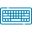# Taylor Series Calculator## Table of Content

Feedback

The Taylor series calculator uses partial sums of an infinite number of terms to approximate the given functions. Our Taylor polynomial calculator determines the series of expansions that come from the function derivatives at a single point.

## What is the Tylor Series?

“Tylor series is an infinite sum given values that are demonstrated as the function derivative at a single point”.

## Formula for Expansion Tylor Series:

The Tylor series is the way to determine any function as a polynomial with an infinite number of terms. The formula taken into service by the Taylor series calculator is as follows:

$$f (x) = \sum\limits_{k=0}^{\infty} \frac{f^{(k)}(a)}{k!}(x - a)^{k}$$

Where:

• f(x) is the function for the nth-order derivative
• a is the central term of the function
• n is the order that indicates the total numbers
• k! Is the factorial

The Taylor Expansion calculator computes the derivative of the sin of x by using the Taylor series expansion formula:

f(x) = sin x = 0

f’(x) = cos (x) = 1

f’’(x) = -sin (x) = 0

f’’’(x) = -cos (x) = -1

f’’’’(x) = sin(x) = 0

### Example:

Evaluate the Tylor series of (x^5)^(1/3) up to order n = 5 and a = 1

Given Data:

3√x^5
n = 5
a = 1

#### Solution:

As we already know the Maclaurin equation to find Taylor series of the polynomials is:

$$f (x) = \sum\limits_{k=0}^{\infty} \frac{f^{(k)}(a)}{k!}(x - a)^{k}$$

$$f (x) ≈ P(x) = \sum\limits_{k=0}^{\infty} \frac{f^{(k)}(a)}{k!}(x - a)^{k} = \sum\limits_{k=0}^{4} \frac{f^{(k)}(a)}{k!}(x - a)^{k}$$

So, what we need to do to get the required polynomial is to evaluate the derivatives, calculate them at a given point, and substitute the results into the formula.

$$f^{(0)}(x) = f(x)= \sqrt{x^{5}}$$

Calculate the function of a point: $$f(1) = 1$$

Step # 1:

Find the 1 derivative by using the Taylor series calculator:

$$f^{(1)}(x) = \left(f^{(0)}(x)\right)^{'}= \left(\sqrt{x^{5}}\right)^{'} = \frac{5 \sqrt{x^{5}}}{3 x}$$

Calculate the 1 derivative at the given point:

$$\left(f(1)\right)^{'} = \frac{5}{3}$$

Step # 2:

Find the 2 derivatives:

$$f^{(2)}(x) = \left(f^{(1)}(x)\right)^{'}= \left(\frac{5 \sqrt{x^{5}}}{3 x}\right)^{'} = \frac{10 \sqrt{x^{5}}}{9 x^{2}}$$

Calculate the 2 derivative at the given point:

$$\left(f(1)\right)^{''} = \frac{10}{9}$$

Step # 3:

Find the 3 derivatives:

$$f^{(3)}(x) = \left(f^{(2)}(x)\right)^{'}= \left(\frac{10 \sqrt{x^{5}}}{9 x^{2}}\right)^{'} = - \frac{10 \sqrt{x^{5}}}{27 x^{3}}$$

The Taylor and Maclaurin series calculator helps to reduce the number of mathematical problems and is used for power flow analysis. So

Calculate the 3 derivative at the given point:

$$\left(f(1)\right)^{'''} = - \frac{10}{27}$$

Step # 4:

Find the 4 derivatives:

$$f^{(4)}(x) = \left(f^{(3)}(x)\right)^{'}= \left(- \frac{10 \sqrt{x^{5}}}{27 x^{3}}\right)^{'} = \frac{40 \sqrt{x^{5}}}{81 x^{4}}$$

Calculate the 4 derivative at the given point:

$$\left(f(1)\right)^{''''} = \frac{40}{81}$$

Step # 5:

Now use the calculated value to obtain the polynomial:

$$f(x) ≈ \frac{1}{0!}(x- (1))^{0} + \frac{\frac{5}{3}}{1!}(x- (1))^{1} + \frac{\frac{10}{9}}{2!}(x- (1))^{2} + \frac{- \frac{10}{27}}{3!}(x- (1))^{3} + \frac{\frac{40}{81}}{4!}(x- (1))^{4}$$

After simplification, we finally get the final answer:

$$f(x)≈P(x)=1+\frac{5 x}{3} - \frac{5}{3}+\frac{5 \left(x - 1\right)^{2}}{9}- \frac{5 \left(x - 1\right)^{3}}{81}+\frac{5 \left(x - 1\right)^{4}}{243}$$

## Working of Taylor Series Calculator:

The Taylor series expansion calculator is used for finding the expansions and series of given functions. It functions only if you provide the following inputs.

What To Do?

• Put the function in the designated field of the tool
• Enter a point to find the tylor series of function
• Insert the value of n for approximation
• Put the optional value to determine the error at that point
• Tap on “Calculate”

What To Get?

An online Taylor polynomials calculator will evaluate the following values if you insert the above values.

• Taylor series after simplification
• The function and derivatives of a given point
• Finds the series of given functions for order n
• Step-by-step results after the simplify

## References:

Wikipedia: Taylor series, Definition, Example, Analytic functions, Approximation error, and convergence, List of Maclaurin series of some common functions, Calculation of Taylor series.### Alan Walker

Studies mathematics sciences, and Technology. Tech geek and a content writer. Wikipedia addict who wants to know everything. Loves traveling, nature, reading. Math and Technology have done their part, and now it's the time for us to get benefits.